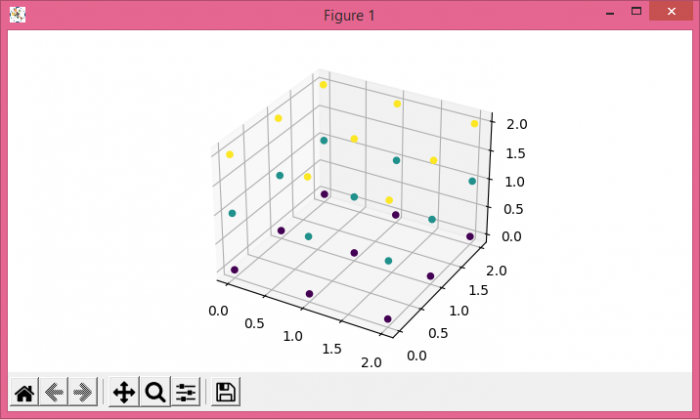# Creating a 3D plot in Matplotlib from a 3D numpy array

To create a 3D plot from a 3D numpy array, we can create a 3D array using numpy and extract the x, y, and z points.

• Create a new figure or activate an existing figure using figure() method.
• Add an '~.axes.Axes' to the figure as part of a subplot arrangement using add_subplot() method.
• Create a random data of size=(3, 3, 3).
• Extract x, y, and z data from the 3D array.
• Plot 3D scattered points on the created axis
• To display the figure, use show() method.

## Example

import numpy as np
from matplotlib import pyplot as plt
plt.rcParams["figure.figsize"] = [7.00, 3.50]
plt.rcParams["figure.autolayout"] = True
fig = plt.figure()
plt.show()